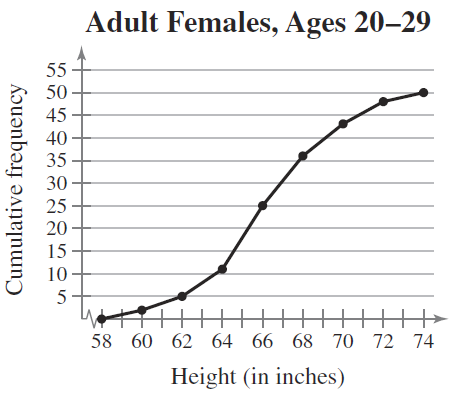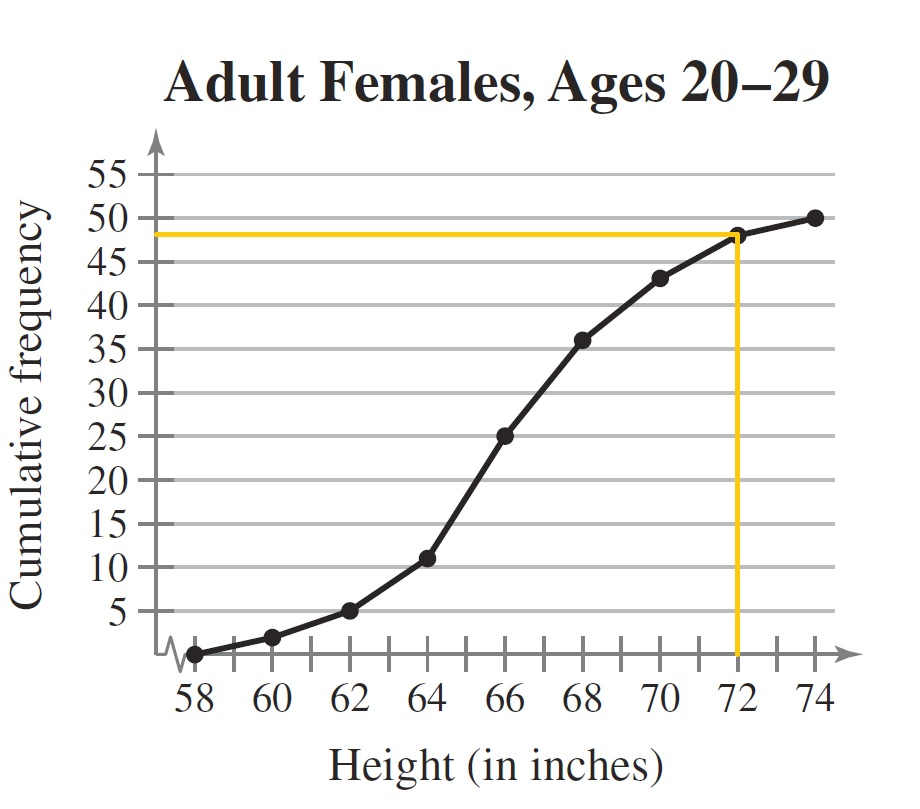×
Get Full Access to Elementary Statistics: Picturing The World - 6 Edition - Chapter 2.1 - Problem 24e
Get Full Access to Elementary Statistics: Picturing The World - 6 Edition - Chapter 2.1 - Problem 24e

×

# Use the ogive In Exercise, to approximate(a) theISBN: 9780321911216 66

## Solution for problem 24E Chapter 2.1

Elementary Statistics: Picturing the World | 6th Edition

• Textbook Solutions
• 2901 Step-by-step solutions solved by professors and subject experts
• Get 24/7 help from StudySoup virtual teaching assistantsElementary Statistics: Picturing the World | 6th Edition

4 5 1 392 Reviews
18
4
Problem 24E

Problem 24E

Use the ogive In Exercise, to approximate

(a) the cumulative frequency for a height of 72 inches.

(b) the height for which the cumulative frequency is 25.

(c) the number of adult females that are between 62 and 66 inches tall.

(d) the number of adult females that are taller than 70 inches.

Exercise

In Exercise, use the ogive to approximate

(a) the number in the sample.

(b) the location of the greatest increase in frequency.Step-by-Step Solution:

Problem 24E

Use the ogive In Exercise, to approximate

(a) the cumulative frequency for a height of 72 inches.

(b) the height for which the cumulative frequency is 25.

(c) the number of adult females that are between 62 and 66 inches tall.

(d) the number of adult females that are taller than 70 inches.

Exercise

In Exercise, use the ogive to approximate

(a) the number in the sample.

(b) the location of the greatest increase in frequency.Step by Step Solution

Step 1 of 4

(a)

From the ogive the cumulative frequency for a height of 72 inches can be determined by drawing a vertical line from 72 inches on the x-axis to the curve and drawing a horizontal line parallel to the x-axis from the meeting point, as shown below. The point at which the horizontal line intersects the vertical axis is, 48.

Therefore the cumulative frequency for a height of 72 inches is 48.Step 2 of 2

##### ISBN: 9780321911216

This textbook survival guide was created for the textbook: Elementary Statistics: Picturing the World , edition: 6. The full step-by-step solution to problem: 24E from chapter: 2.1 was answered by , our top Statistics solution expert on 08/25/17, 09:43AM. This full solution covers the following key subjects: adult, inches, frequency, females, exercise. This expansive textbook survival guide covers 66 chapters, and 3049 solutions. Elementary Statistics: Picturing the World was written by and is associated to the ISBN: 9780321911216. The answer to “Use the ogive In Exercise, to approximate(a) the cumulative frequency for a height of 72 inches.________________(b) the height for which the cumulative frequency is 25.________________(c) the number of adult females that are between 62 and 66 inches tall.________________(d) the number of adult females that are taller than 70 inches.ExerciseIn Exercise, use the ogive to approximate(a) the number in the sample.________________(b) the location of the greatest increase in frequency.Adult Females, Ages 20–29” is broken down into a number of easy to follow steps, and 71 words. Since the solution to 24E from 2.1 chapter was answered, more than 735 students have viewed the full step-by-step answer.

Unlock Textbook Solution